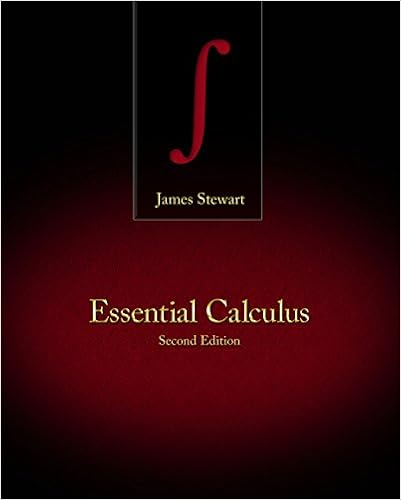# Practice Exam 2 Solutions - Math 202 Practice exam 2 Show...

• 3

This preview shows page 1 - 2 out of 3 pages.

##### We have textbook solutions for you!
The document you are viewing contains questions related to this textbook.The document you are viewing contains questions related to this textbook.
Chapter 12 / Exercise 8
Essential Calculus
StewartExpert Verified
Math 202 Practice exam 2 Show all work in a clear and concise manner to get maximum credit. Circle your answers. 1. Consider a wire in space parametrized by ~ c ( t ) = < 3 t, - t 2 , 2 t 2 >, 0 t 1 . a. (10 points) Set up, but do not evaluate , the integral for the arc length of the wire.
b. (10 points) Suppose the density of the wire at a point ( x, y, z ) is ρ ( x, y, z ) = x . Find the mass of the wire.
2. (15points) Let ~ F ( x, y, z ) = < e x yz, e x z + 2 yz, e x y + y 2 + 1 > . Evaluate R ~ c ~ F · d~s where ~ c ( t ) = < cos t, sin t, t >, 0 t π 2 . Hint: look for a shortcut. 3. Consider the double integral R 1 0 (
##### We have textbook solutions for you!
The document you are viewing contains questions related to this textbook.The document you are viewing contains questions related to this textbook.
Chapter 12 / Exercise 8
Essential Calculus
StewartExpert Verified
•••